true

Soumya HSFeatured

# Soumya HS## Math Tutor

7 Students taught

Referral Discount: Get ₹ 500 off when you make a payment to start classes. Get started by Booking a Demo.

Details verified of Soumya HS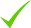IdentityEducation

Know how UrbanPro verifies Tutor details

Identity is verified based on matching the details uploaded by the Tutor with government databases.

## Overview

I communicate effectively with students. My strength is my knowledge in subject. I teach very effectively. All my tuition students got more than 75% in PUC exam and good ranking in CET and comed-k.

English

## Education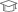St Aloysius college, mangalore 2014

Master of Science (M.Sc.)

## Verified Info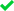ID VerifiedEducation VerifiedPhone VerifiedEmail Verified

Report this Profile

Is this listing inaccurate or duplicate? Any other problem?

Type the letters as shown below *

Please enter the letters as show below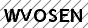Class 11 Tuition

Class LocationOnline (video chat via skype, google hangout etc)Student's HomeTutor's Home

Years of Experience in Class 11 Tuition

6

Board

CBSE, ISC/ICSE, State

ISC/ICSE Subjects taught

Mathematics

CBSE Subjects taught

Mathematics

Taught in School or College

Yes

State Syllabus Subjects taught

Mathematics

## Reviews

this is test message this is test message this is test message this is test message this is test message this is test message this is test message

No Reviews yet! Be the first one to Review

## FAQs

1. Which school boards of Class 12 do you teach for?

CBSE, ISC/ICSE and State

2. Have you ever taught in any School or College?

Yes

3. Which classes do you teach?

I teach Class 10 Tuition, Class 11 Tuition, Class 12 Tuition and Class I-V Tuition Classes.

4. Do you provide a demo class?

Yes, I provide a free demo class.

5. How many years of experience do you have?

I have been teaching for 6 years.

## Answers by Soumya HS (2)

If f(-x)=f(x) for all x belongs to domain of f then f is called an even function. Eg: f(x)=sin^2x f(-x)=sin^2(-x) =(-sinx)^2 =sin^2x =f(x) Therefore f(x) is even. If f(-x)=-f(x) for all x belongs to domain of f then f is called an odd function. Eg:f(x)=... ...more
If f(-x)=f(x) for all x belongs to domain of f then f is called an even function. Eg: f(x)=sin^2x f(-x)=sin^2(-x) =(-sinx)^2 =sin^2x =f(x) Therefore f(x) is even. If f(-x)=-f(x) for all x belongs to domain of f then f is called an odd function. Eg:f(x)= sin^7x f(-x)=sin^7(-x) =sin(-x)^7 =(-sinx)^7 =-sin^7 =-f(x) Therefore f(x) is odd.
Dislike Bookmark

I only teach mathematics. I take Rs.500 per hour.
Dislike Bookmark
Class 11 Tuition 5.0

Class LocationOnline (video chat via skype, google hangout etc)Student's HomeTutor's Home

Years of Experience in Class 11 Tuition

6

Board

CBSE, ISC/ICSE, State

ISC/ICSE Subjects taught

Mathematics

CBSE Subjects taught

Mathematics

Taught in School or College

Yes

State Syllabus Subjects taught

Mathematics

Class 12 Tuition 5.0

Class LocationOnline (video chat via skype, google hangout etc)Student's HomeTutor's Home

Years of Experience in Class 12 Tuition

6

Board

CBSE, ISC/ICSE, State

ISC/ICSE Subjects taught

Mathematics

CBSE Subjects taught

Mathematics

Experience in School or College

I have 5 years of teaching experience for PUC.

Taught in School or College

Yes

State Syllabus Subjects taught

Mathematics

Class 10 Tuition 5.0

Class LocationOnline (video chat via skype, google hangout etc)Student's HomeTutor's Home

Years of Experience in Class 10 Tuition

6

Board

State, CBSE, ICSE

CBSE Subjects taught

Mathematics

ICSE Subjects taught

Mathematics

Taught in School or College

Yes

State Syllabus Subjects taught

Mathematics

Class I-V Tuition 5.0

Class Location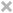Online (video chat via skype, google hangout etc)Student's HomeTutor's Home

Years of Experience in Class I-V Tuition

5

Fees

₹ 600 per hour

Board

State, ICSE, CBSE

CBSE Subjects taught

Mathematics

ICSE Subjects taught

Mathematics

Taught in School or College

Yes

State Syllabus Subjects taught

Mathematics

this is test message this is test message this is test message this is test message this is test message this is test message this is test message

No Reviews yet! Be the first one to Review

## Answers by Soumya HS (2)

If f(-x)=f(x) for all x belongs to domain of f then f is called an even function. Eg: f(x)=sin^2x f(-x)=sin^2(-x) =(-sinx)^2 =sin^2x =f(x) Therefore f(x) is even. If f(-x)=-f(x) for all x belongs to domain of f then f is called an odd function. Eg:f(x)=... ...more
If f(-x)=f(x) for all x belongs to domain of f then f is called an even function. Eg: f(x)=sin^2x f(-x)=sin^2(-x) =(-sinx)^2 =sin^2x =f(x) Therefore f(x) is even. If f(-x)=-f(x) for all x belongs to domain of f then f is called an odd function. Eg:f(x)= sin^7x f(-x)=sin^7(-x) =sin(-x)^7 =(-sinx)^7 =-sin^7 =-f(x) Therefore f(x) is odd.
Dislike Bookmark

I only teach mathematics. I take Rs.500 per hour.
Dislike Bookmark

Soumya HS describes herself as Math Tutor. She conducts classes in Class 10 Tuition, Class 11 Tuition and Class 12 Tuition. Soumya is located in Sunkadakatte, Bangalore. Soumya takes at students Home and Regular Classes- at her Home. She has 6 years of teaching experience . Soumya has completed Master of Science (M.Sc.) from St Aloysius college,mangalore in 2014. She is well versed in Kannada and English.

•• Want to learn from Soumya HS?

• Contact Now
X

Recommended Profiles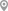Krishnarajapura, BangaloreSunkadakatte, BangaloreBellandur, BangaloreAshok Nagar, Banashankari , BangaloreBtm Layout 2nd Stage, BangaloreSunkadakatte, Bangalore

X

Let us shortlist and give the best tutors and institutes.

or

Send Enquiry to Soumya HS

Let Soumya HS know you are interested in their class